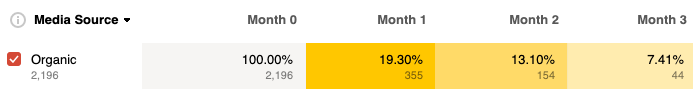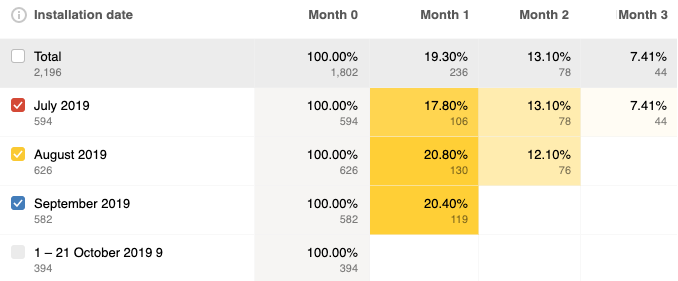# How retention is calculated?

In the example:
• Date range is July 1 — October 21.
• Grouping by sources is enabled.
• The time range is divided into months.
• Retention metrics is selected.Example of calculating the percentage of users who returned in the first month after installation:The report displays 19.30%. The resulting numbers differ because the interface displays the number of all returned users grouped by the partner, and the percentage of returns is calculated only for the completed time interval.For example, it's the 21 of October. For installations made in September, the first month (October) is not over yet. So September is not included into the calculation of the final value of the first month. Therefore, to calculate the percentage of returns in the first month, you need to sum only the values of the zero months, which values are taken into account.

To calculate the percentage of returned users in the first month:

1. Turn on grouping by installation date.
2. Enable segmentation by the appropriate installation source.
3. Divide the total number of returned users in the first month by the total number of installations in July and August:In the report, the value of 19.34 is rounded to a decimal place.